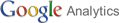﻿### The World Of Energy

 HydraulicsMonday, 27 September 2010 17:57 1. ABOUT SOME ISSUES OF ANALYSIS OF EQUATIONS OF DYNAMICS OF COMPOSITE WATER-SOIL MIXTUREL. MINKIN (Israel)Equation system of water-soil mixture motion proposed by Professor T. G. Voynich-Sianozhenski is reviewed. Some solutions of the equation system of linear and nonlinear statements elaborated by the author are provided. Methods of reducing them to Burgers equation system and then to general positions of heat conductivity are presented.Calculations for estimating wave parameters according to various methods are performed. The first method includes numeric solution of the equation system of the motion according to the method of characteristics earlier worked out by the author, the second – approximate solution of the system according to Dressler model, the third is based on application of perturbation method and finally, the forth uses both reduction of basic equations to Burgers equation and then, as a consequence, to the equation of heat conductivity and the calculations of ready relations provided in the book by James Aussem “linear and nonlinear waves” (1977).Last Updated on Saturday, 02 October 2010 19:09